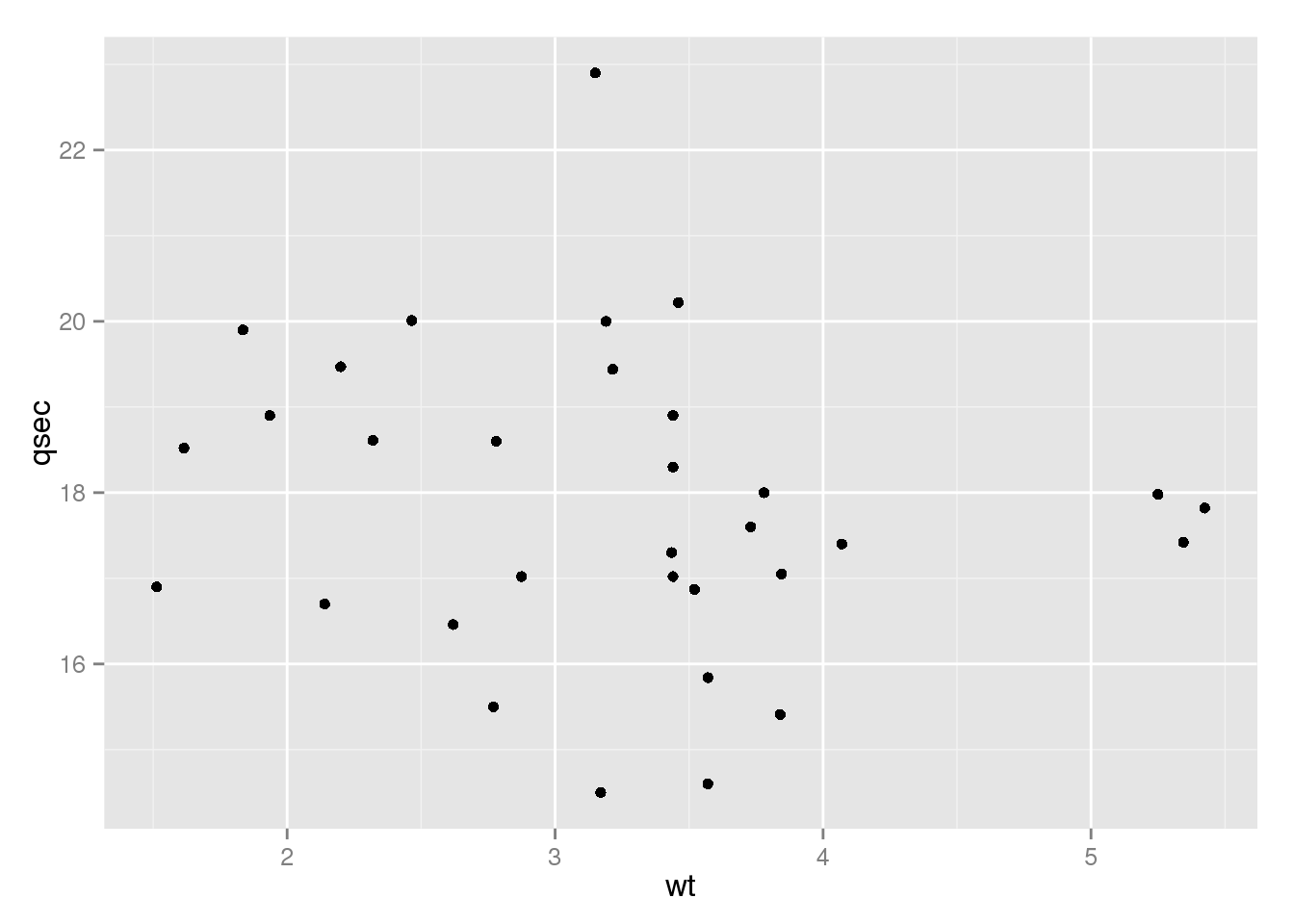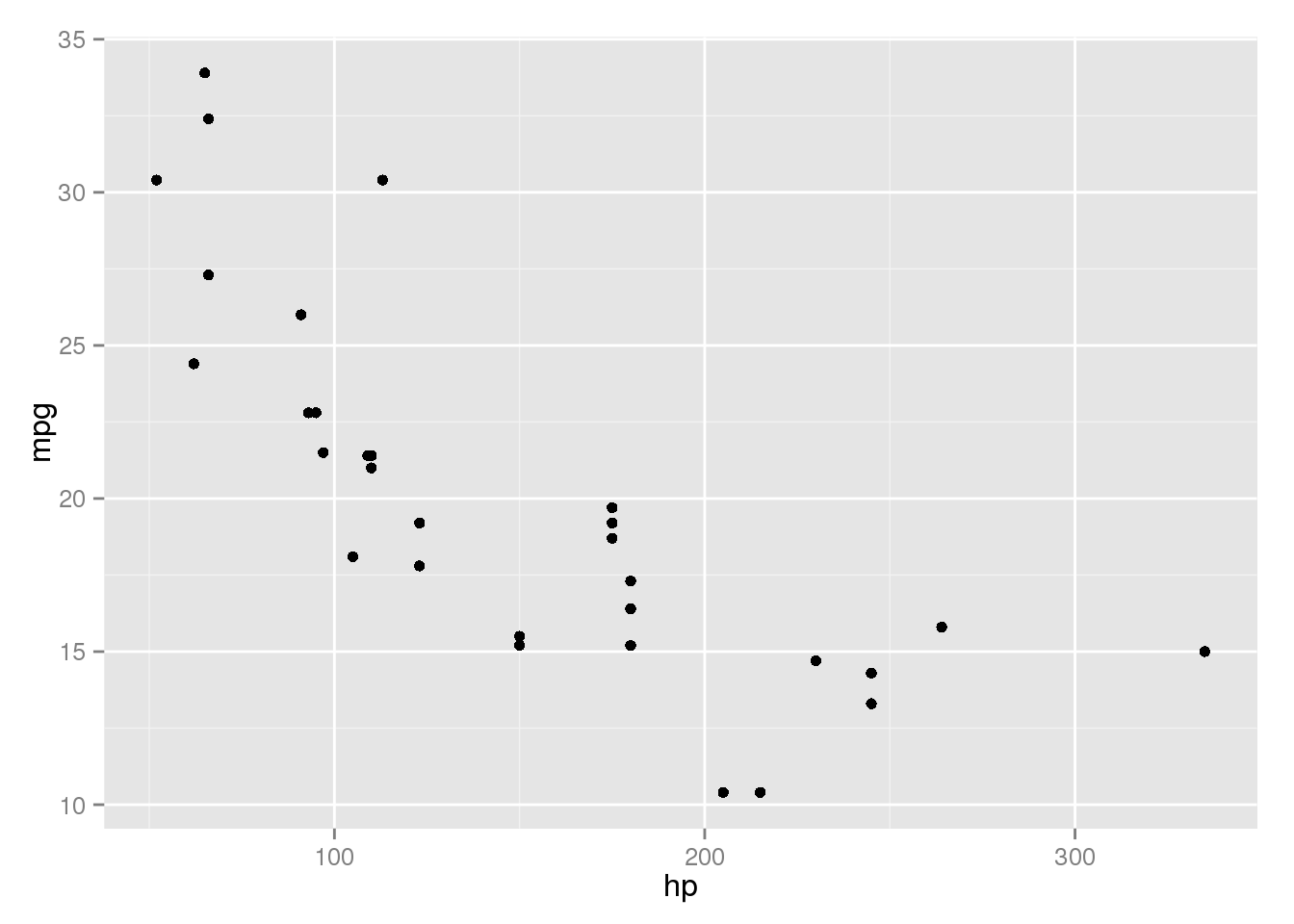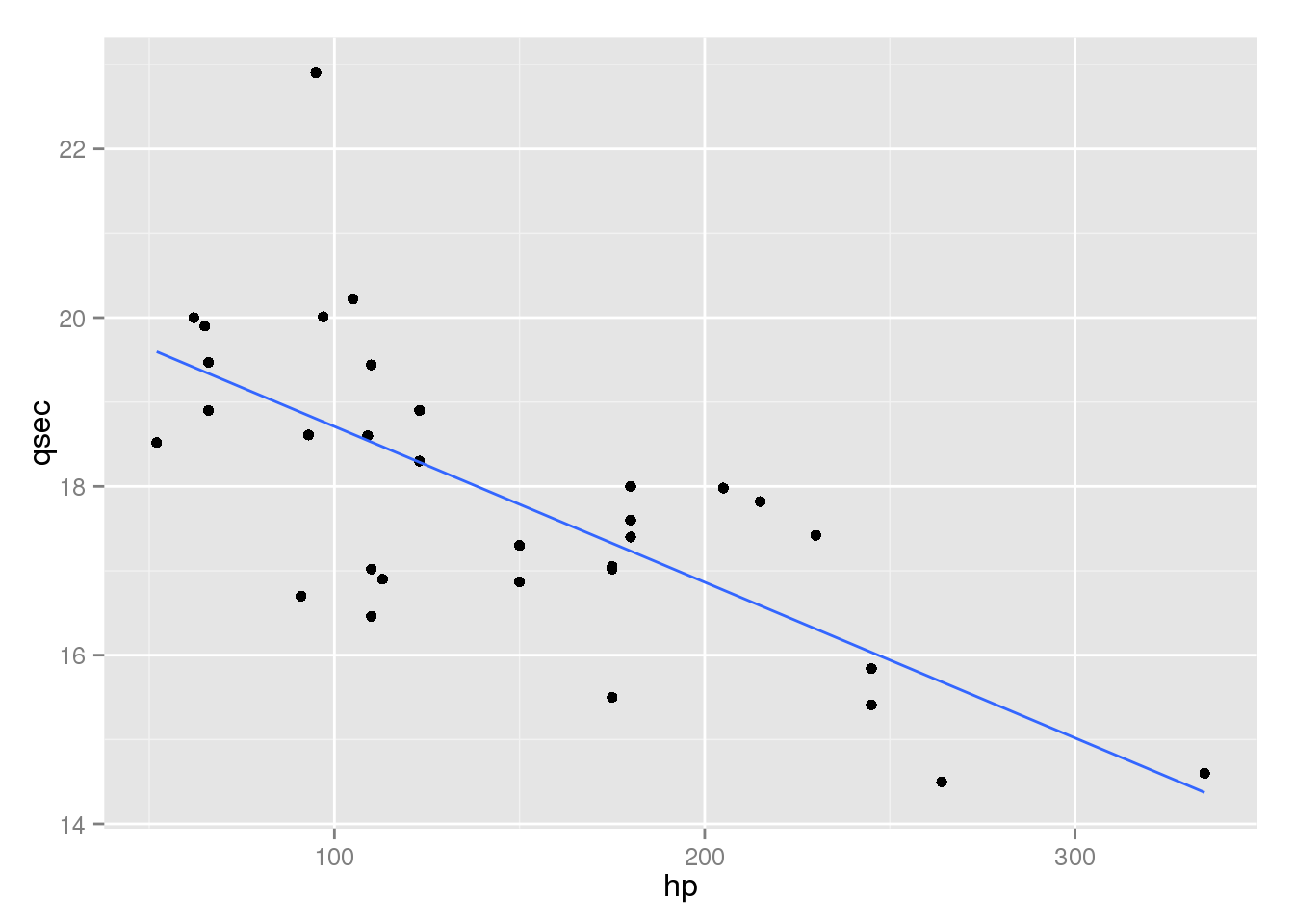## Overview

In this lab, we will continue examining the Motor Trend Cars data set.

The data was extracted from the 1974 Motor Trend US magazine, and comprises fuel consumption and 10 aspects of automobile design and performance for 32 automobiles (1973–74 models).

Variable Description
mpg Miles/(US) Gallon
cyl Number of Cylinders
disp Displacement (cu.in.)
hp Gross horsepower
wt Weight (lb/1000)
qsec 1/4 mile time (seconds)
vs V or Straight Configuration Engine
am Transmission Type (auto/manual)

## Load the Data in StatCrunch

A Tab Separated File (.tsv) containing the data can be found here:

1. In StatCrunch, use the menus Data $$\to$$ Load $$\to$$ From File $$\to$$ On the web.
2. Copy and Paste the address given above into WWW Address
3. Click Load File at the bottom of the page.

## Complete the following problems on a separate piece of paper. Read these instructions carefully.

• Hand in your answers at the end of lab – make sure to include you name and the lab number. Instructions are included for making graphs and finding the necessary statistics for each problem.
• Each question will be worth two points, and you can receive partial credit for incorrect answers if your process was correct.
• Write your final answer as a sentence and include all steps you used to get there, otherwise you will receive partial credit.
• When sketching scatterplots, you do not need to include every point, just make sure you show the overall pattern and any outliers, if they exist. Keep in mind that standard notation for naming scatterplots is $$Y$$ vs. $$X$$.
• When performing calculations, keep intermediate steps rounded to four decimal places, and round your final answer to two decimal places.

Correlation:

• Stat $$\to$$ Summary Stats $$\to$$ Correlation

Scatterplots:

• Graph $$\to$$ Scatter Plot $$\to$$ Select $$X$$ and $$Y$$

Regression:

• Stat $$\to$$ Regression $$\to$$ Simple Linear $$\to$$ Select $$X$$ and $$Y$$
• Note that this output also includes correlation information and a scatterplot with fitted line
• The scatterplot with fitted line can be found by clicking the arrow at the bottom of the output window.

### Problems:

1. Make a scatterplot of quarter mile time (qsec) vs. weight (wt). Sketch the graph.
library(ggplot2)
header = TRUE, sep = "\t")
ggplot(mtcars2, aes(x = wt, y = qsec)) + geom_point()1. Does there appear to be a strong linear relationship between qsec and wt? Include any relevant statistics.
with(mtcars2, cor(qsec, wt))
##  -0.1747159

The scatter plot and correlation coefficient of $$r = -0.17$$ suggest there is an extremely weak negative or no linear association between quarter mile time and weight.

1. Fit the regression line that that predicts engine size (disp) with weight (wt). Report the line equation and interpret the slope and intercept in the context of the data set; make sure to include units in your description. (keep in mind that weight is measured in thousands of pounds).
summary(lm(disp ~ wt, mtcars2))
##
## Call:
## lm(formula = disp ~ wt, data = mtcars2)
##
## Residuals:
##    Min     1Q Median     3Q    Max
## -88.18 -33.62 -10.05  35.15 125.59
##
## Coefficients:
##             Estimate Std. Error t value Pr(>|t|)
## (Intercept)  -131.15      35.72  -3.672 0.000933 ***
## wt            112.48      10.64  10.576 1.22e-11 ***
## ---
## Signif. codes:  0 '***' 0.001 '**' 0.01 '*' 0.05 '.' 0.1 ' ' 1
##
## Residual standard error: 57.94 on 30 degrees of freedom
## Multiple R-squared:  0.7885, Adjusted R-squared:  0.7815
## F-statistic: 111.8 on 1 and 30 DF,  p-value: 1.222e-11

Our regression line is: $$\widehat{disp} = -131.15 - 112.48wt$$. This suggests that engine size increases 112.48 cubic inches for every additional thousand pounds of car weight, on average.

The y-intercept tells us that we would predict a car weighing zero pounds having an engine displacement of -131.15 $$in^3$$. Since a car cannot weigh zero pounds, and volume cannot be negative, this is not interpretable.

1. Report the $$R^2$$ statistic for this model. What does it tell us about the relationship?

For this model, $$R^2 = 0.7885$$, telling us that 78.85% of the variability in engine size is accounted for by the weight of the car.

1. Sketch a scatterplot of mpg vs. hp. Describe the relationship, making sure to include any relevant statistics. Would linear regression be appropriate?
ggplot(mtcars2, aes(x = hp, y = mpg)) + geom_point()with(mtcars2, cor(hp, mpg))
##  -0.7761684

The pattern in the scatterplot and correlation coefficient of $$r - 0.78$$ suggest a moderately strong negative linear relationship between fuel efficiency and horsepower. Because of this, a linear model would appropriately describe the relationship.

1. Fit the Least Squares Regression line for predicting fuel efficiency (mpg) from horsepower (hp). Report the line equation and interpret the slope and intercept in the context of the data set; make sure to include units in your description.
summary(lm(mpg ~ hp, mtcars2))
##
## Call:
## lm(formula = mpg ~ hp, data = mtcars2)
##
## Residuals:
##     Min      1Q  Median      3Q     Max
## -5.7121 -2.1122 -0.8854  1.5819  8.2360
##
## Coefficients:
##             Estimate Std. Error t value Pr(>|t|)
## (Intercept) 30.09886    1.63392  18.421  < 2e-16 ***
## hp          -0.06823    0.01012  -6.742 1.79e-07 ***
## ---
## Signif. codes:  0 '***' 0.001 '**' 0.01 '*' 0.05 '.' 0.1 ' ' 1
##
## Residual standard error: 3.863 on 30 degrees of freedom
## Multiple R-squared:  0.6024, Adjusted R-squared:  0.5892
## F-statistic: 45.46 on 1 and 30 DF,  p-value: 1.788e-07

Our regression line is: $$\widehat{mpg} = 30.0987 - 0.0682hp$$. On average, a car will lose 0.07 mpg for every increase in horsepower.

The y-intercept of 30.10 suggests that a car with zero horsepower has a fuel efficiency of 30.1 mpg. Since a car with zero horsepower wouldn’t move at all, this coefficient is only useful for making predictions and drawing the line, but is not interpretable.

1. Find the expected fuel efficiency for the Maserati Bora based just on its horsepower (show your work). Describe how well the prediction matches with the actual value using the residual.

\begin{align} \widehat{mpg} &= 30.0987 - 0.0682hp\\ \widehat{mpg} &= 30.0987 - 0.0682(335)\\ \widehat{mpg} &= 30.0987 - 22.847\\ \widehat{mpg} &= 7.2517\\ \end{align}

Based just on its horsepower, we would expect the Maserati Bora to have a fuel efficiency of 7.25.

\begin{align} residual &= mpg - \widehat{mpg}\\ residual &= 15 - 7.2517\\ residual &= 7.7483\\ \end{align}

The Maserati has a residual of 7.75, so it has much better fuel efficiency than expected.

1. Fit a linear model which predicts quarter mile time (qsec) from horsepower (hp). Report the line equation and interpret the slope and intercept in the context of the data set; make sure to include units in your description.
(mod <- summary(lm(qsec ~ hp, mtcars2)))
##
## Call:
## lm(formula = qsec ~ hp, data = mtcars2)
##
## Residuals:
##     Min      1Q  Median      3Q     Max
## -2.1766 -0.6975  0.0348  0.6520  4.0972
##
## Coefficients:
##              Estimate Std. Error t value Pr(>|t|)
## (Intercept) 20.556354   0.542424  37.897  < 2e-16 ***
## hp          -0.018458   0.003359  -5.495 5.77e-06 ***
## ---
## Signif. codes:  0 '***' 0.001 '**' 0.01 '*' 0.05 '.' 0.1 ' ' 1
##
## Residual standard error: 1.282 on 30 degrees of freedom
## Multiple R-squared:  0.5016, Adjusted R-squared:  0.485
## F-statistic: 30.19 on 1 and 30 DF,  p-value: 5.766e-06

The linear model to predict quarter second time from horsepower is: $$\widehat{qsec} = 20.5564 - 0.0185hp$$. On average, an increase of one horsepower improves a cars quarter mile time by 0.02 seconds.

The y-intercept suggests that a car with no horsepower could complete a quarter mile drag race in 20.56 seconds, which doesn’t make sense. Because of this, the y-intercept is only useful for drawing the line or making predictions, not in describing the real world.

1. What percent of the variability in quarter mile time is accounted for by horsepower?

Since we have $$R^2 = 0.5016$$, 50.16% of the variability in quarter mile times is accounted for by horsepower. Other factors, such as the transmission type, weight, etc. probably play a role.

1. Which car has the highest residual for this linear model (you can get information about a point by hovering over it with your mouse)? What is the residual?
ggplot(mtcars2, aes(x = hp, y = qsec)) + geom_point() + geom_smooth(method = "lm", se = FALSE)mtcars2[which.max(residuals(mod)), ]
##        car  mpg cyl  disp hp   wt qsec vs     am
## 9 Merc 230 22.8   4 140.8 95 3.15 22.9  S manual

The car furthest from the line is is the Mercedes 230, which has 95 horsepower and a quarter mile time of 22.9 seconds.

\begin{align} \widehat{qsec} &= 20.5564 - 0.0185hp\\ \widehat{qsec} &= 20.5564 - 0.0185(95)\\ \widehat{qsec} &= 20.5564 - 1.7575\\ \widehat{qsec} &= 18.7989\\ \end{align}

The model predicts that the Mercedes 230 would finish the quarter mile in 18.80 seconds based on its horsepower alone. The residual is:

$qsec - \widehat{qsec} = 20.5564 - 18.7989 = 1.7575$

The residual of 1.76 tells us that the Mercedes 230 is 1.76 seconds slower than we’d expect.